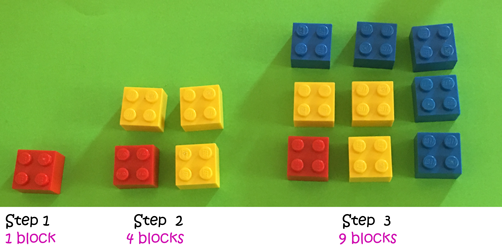Pull Down & Release to Refresh

# Definition of Number PatternA number pattern is a list of numbers that follow a given rule.

For example, the sequence of numbers $1,2,4,8,16,\dots$ doubles each time.

Another example is the sequence of numbers $5,15,25,35,\dots$, which starts at $5$ and jumps by $10$ each time.

Each number in the number pattern represented by the blocks in the picture is a square number. The first one is equal to one squared, the second one is equal to two squared, the third one is equal to three squared, and so on.

### Description

The aim of this dictionary is to provide definitions to common mathematical terms. Students learn a new math skill every week at school, sometimes just before they start a new skill, if they want to look at what a specific term means, this is where this dictionary will become handy and a go-to guide for a student.

### Audience

Year 1 to Year 12 students

### Learning Objectives

Learn common math terms starting with letter N

Author: Subject Coach
You must be logged in as Student to ask a Question.